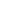# Free CBSE Class 8 Maths Data handling Worksheets

Download free printable Data handling Worksheets to practice. With thousands of questions available, you can generate as many Data handling Worksheets as you want.

## Sample CBSE Class 8 Maths Data handling Worksheet Questions

1.

The graph below shows the hours Sarah worked each day of the week. On which day Sarah worked the most?1. Monday 2. Friday 3. Thursday 4. Tuesday
2.

The graph below shows the number of books a class read each month. Which month had the least number of books read?3.

From the following graph determine the total distance travelled.1. 1500 m 2. 1125 m 3. 2455 m 4. 1200 m
4.

Following pie chart shows the percentage of viewers watching different types of TV channels.What is sector angle (in degree) which represents the viewer of Informative channel?

1. 45 2. 90 3. 72 4. 36
5.

Some children were asked to name their favorite flavor of ice cream. The following pie chart shows the information about their answers.If number of children are 3600. Find the number of children who named strawberry as their favorite.

1. 450 2. 900 3. 1050 4. 1200
6.

There are 2000 students in a school. The following pie chart shows the distribution of enrollment of students in various sports.Find the number of students enrolled in chess

7.

The following histogram depicts the marks obtained by 45 students of a class in Maths test.Answer the following questions based on above histogram:

1).  How many students obtained 20 or more marks but less than 35?

2).  How many students got less than 15 marks?

3).  If a first division is equal to 25 or more marks, how many students obtained this division?

4).  In which class-interval of marks the number of students were maximum?

8.

The following histogram shows the height of 42 students in the class.1). How many students are having height less than 155 cm?

2). How many students have height equal to 160 or more?

3). How many students are having height less than 170 cm?

9.1).  What is the number of students in the marks group of 50–60?

2).  In which group the number of students are maximum?

3).  In which group the number of students are minimum?

10.

The following bar graph shows the result of class XII of a school:Answer the following questions based on the above bar graph:

1). In which year the increase in the result was maximum?

2). In which year the result of Class XII falls?

3). in which year the result of Class XII was minimum?

## Find more Data handling Worksheets

Worksheets by UrbanPro

Our worksheets are designed to help students explore various topics, practice skills and enrich their subject knowledge, to improve their academic performance. Designed by Experts who have extensive experience and expertise in teaching a subject, these worksheets will improve your child's problem-solving skills and subject knowledge in a fun and interactive manner.
Check out our free customized worksheets across school boards, grades, subjects and levels of subject knowledge. You can download, print and share these worksheets with anyone, anywhere, anytime!

Get a custom worksheet to practice!

Select your topic & see the magic.

subjectSelect Chapter(s)

Chapters & Subtopics